# 删除系统中的重复文件夹

## 1948. 删除系统中的重复文件夹 (Hard)

• 例如，`["one", "two", "three"]` 表示路径 `"/one/two/three"`

• 例如，下面文件结构中的文件夹 `"/a"``"/b"` 相同。它们（以及它们的子文件夹）应该被 全部 标记为待删除：
• `/a`
• `/a/x`
• `/a/x/y`
• `/a/z`
• `/b`
• `/b/x`
• `/b/x/y`
• `/b/z`
• 然而，如果文件结构中还包含路径 `"/b/w"` ，那么文件夹 `"/a"``"/b"` 就不相同。注意，即便添加了新的文件夹 `"/b/w"` ，仍然认为 `"/a/x"``"/b/x"` 相同。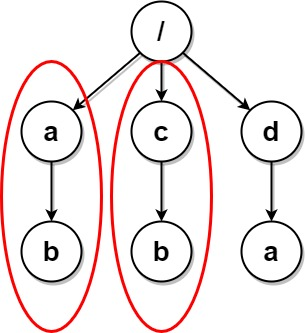```输入：paths = [["a"],["c"],["d"],["a","b"],["c","b"],["d","a"]]

```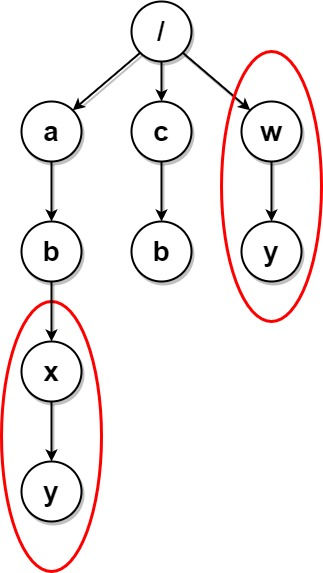```输入：paths = [["a"],["c"],["a","b"],["c","b"],["a","b","x"],["a","b","x","y"],["w"],["w","y"]]

```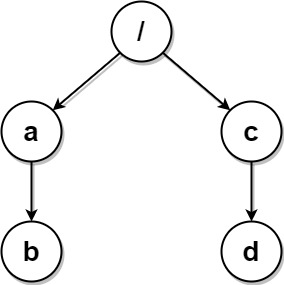```输入：paths = [["a","b"],["c","d"],["c"],["a"]]

```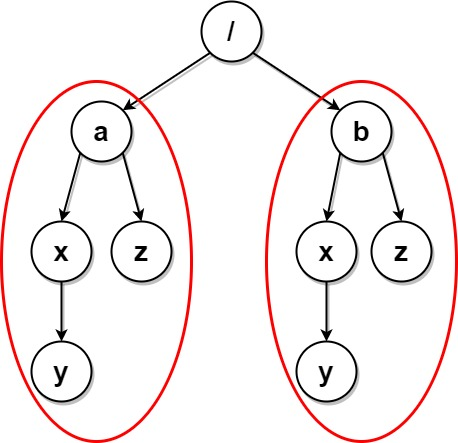```输入：paths = [["a"],["a","x"],["a","x","y"],["a","z"],["b"],["b","x"],["b","x","y"],["b","z"]]

```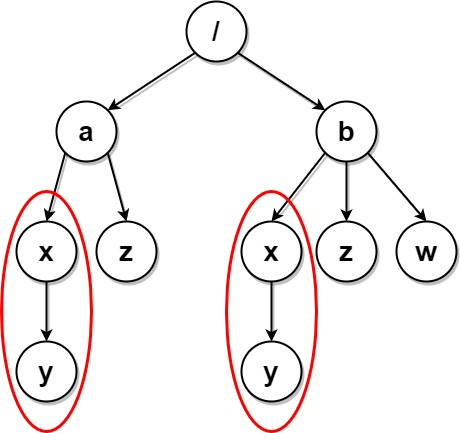```输入：paths = [["a"],["a","x"],["a","x","y"],["a","z"],["b"],["b","x"],["b","x","y"],["b","z"],["b","w"]]

```

• `1 <= paths.length <= 2 * 104`
• `1 <= paths[i].length <= 500`
• `1 <= paths[i][j].length <= 10`
• `1 <= sum(paths[i][j].length) <= 2 * 105`
• `path[i][j]` 由小写英文字母组成
• 不会存在两个路径都指向同一个文件夹的情况
• 对于不在根层级的任意文件夹，其父文件夹也会包含在输入中

### 相关话题

[字典树] [数组] [哈希表] [字符串] [哈希函数]